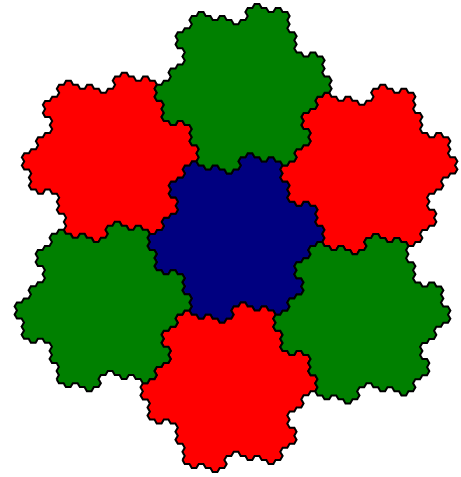# Nested lattices

1, 240, 2160, 6720, 17520, 30240, 60480, 82560, 140400, …

These terms count the number of points at distance$\sqrt{2n}$ from the origin in the E8 lattice, a highly symmetric arrangement of points which Maryna Viazovska recently (in 2016) proved is the densest way to pack spheres in 8-dimensional space.

Even more recently, Warren D. Smith noticed a rather exceptional numerical coincidence — the sum of the first three terms in this sequence is a perfect fourth power:

1 + 240 + 2160 = 2401 = 7^4

Is this merely a coincidence? To begin with, we’ll look at the E8 lattice through a different lens: as a subset not of$\mathbb{R}^8$, but of complex (alas, not projective) 4-space,$\mathbb{C}^4$. Specifically, recall the Eisenstein integers, the ring of complex numbers generated by a cube root of unity:These points have been 3-coloured according to whether the Eisenstein integer is congruent (modulo$\sqrt{-3}$ ) to 0, +1, or −1. The E8 lattice is then concisely expressible as the set of points (w, x, y, z) where:

• each coordinate is an Eisenstein integer;
• the colours of the four coordinates form a codeword in the tetracode.

The tetracode is a 2-dimensional subspace of the 4-dimensional vector space$\mathbb{F}_3^4$. A point$(w, x, y, z) \in \mathbb{F}_3^4$ belongs to the tetracode if and only if w = y − x = z − y = x − z. Equivalently, the coordinates are of the form (d, a, a + d, a − d).

The real E8 lattice has a symmetry group of order 696729600; this complex E8 lattice has more structure (complex scalar multiplication) which reduces the symmetry group to order 155520. This extra structure is what we need to explain Warren’s coincidence.

Now that we have defined the E8 lattice as a complex 4-dimensional lattice, consider scalar multiplication by 3 + ω. Returning to an 8-dimensional real viewpoint, this linear map corresponds to composing a rotation by a scaling by sqrt(7), and therefore has a determinant of sqrt(7)^8 = 2401. The image of the E8 lattice under this map is a sublattice, geometrically similar to the original E8 lattice, and the Voronoi cells induced by this sublattice each consist of 2401 points from the original lattice (namely one central point, surrounded by an inner shell of 240 points and an outer shell of 2160 points).

### Linear subspaces and positional number systems

If we sum the first three terms of the theta series of the E6 lattice, 1 + 72 + 270, we get exactly 7^3. And similarly for the D4 lattice, 1 + 24 + 24 = 7^2. For the A2 (hexagonal) lattice, we have 1 + 0 + 6 = 7^1. These correspond to taking 3-, 2-, and 1-dimensional (complex) linear subspaces of the Voronoi partition described above.

The latter is particularly easy to describe: every Eisenstein integer z can be uniquely expressed in the form (3 + ω)q + r, where q is an Eisenstein integer and r belongs to the set {0, 1, ω, ω², 1, ω, ω²} consisting of zero together with the sixth roots of unity. We can decompose q in the same manner, and continue recursively; this is tantamount to writing an Eisenstein integer in a positional number system with radix 3 + ω and the seven digits {0, 1, ω, ω², 1, ω, ω²}.

If we allow digits after the ‘decimal point’, we can express any complex number in this positional number system. The set of numbers which ’round to zero’ after discarding the digits after the decimal point form the Gosper island, a self-similar set with a fractal boundary:There are two different principal cube roots of unity, and each one yields a different (albeit isomorphic) positional number system for complex numbers.

The Hurwitz integral quaternions form a scaled copy of the D4 lattice, and we similarly obtain a positional number system for the quaternions with radix 3 + ω and 49 different digits: the Hurwitz integral quaternions with squared norm at most 2. There are 8 principal cube-roots of unity, and each one determines a positional number system for quaternions. It’s worth commenting that this isn’t the only nice* number system for integral quaternions: radix-(2 + i) with 25 digits (the Hurwitz integers of norm at most 1) also works.

*where ‘nice’ is defined to mean that the digit set consists of all integers with norm <= r for some value of r.

Finally, the Cayley integer octonions form a scaled copy of the E8 lattice, and there are 56 principal cube-roots of unity. Any one of these results in a positional number system for the octonions (which is well-defined despite the non-associativity of the octonions, since any pair of octonions together generate an associative algebra) with radix 3 + ω and 2401 different digits: the integer octonions with squared norm at most 2. We’ll call this number system ‘Warrenary’ after its discoverer. Unlike in the real, complex, and quaternionic cases, Warrenary is the unique positional number system for the octonions satisfying the aforementioned niceness property.

There is no analogue in six dimensions: normed division algebras only exist in dimensions 1, 2, 4, and 8, so there is no positional number system corresponding to the recursive nesting of E6 lattices.

Disappointingly, there appears not to be any similarly elegant lattice nestings for higher-dimensional lattices beyond D4, E6 and E8, such as the 24-dimensional Leech lattice (in particular, no early initial segment of the theta series yields a perfect 12th power as its sum). As such, the recursive nesting of E8 lattices is quite exceptional indeed.

1.J Gregory Moxness says: